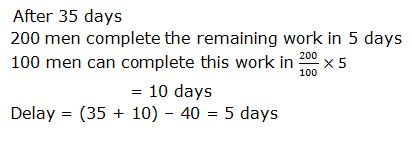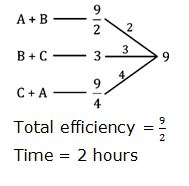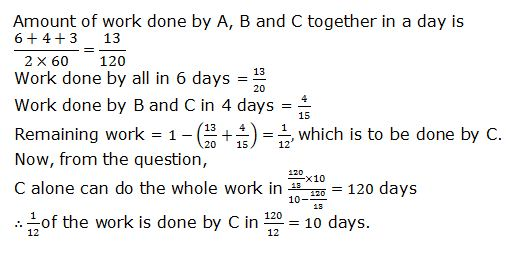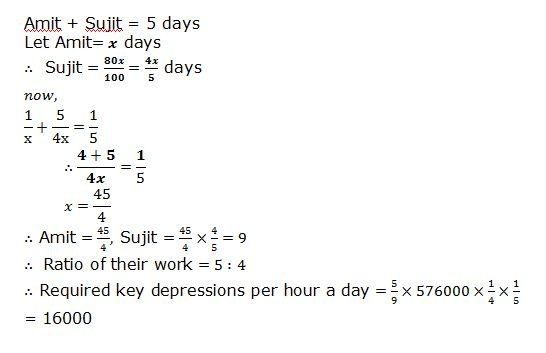# gkrecall

## Breaking

SBI Clerk and SBI PO Prelims exam are going to be held in the upcoming months. We have already provided you with the PDFs of many topics of Quantitative Aptitude like Simplification/Approximation, Number Series,datainterpretation.important Arithmetic questions to prepare for SBI Clerk and PO Pre exam. Practicing these questions will help you to know about the level of the questions. To increase your speed and accuracy, enhance your calculations.

START TEST

1.
x² – 44x + 448 = 0
y² – 28y + 195 = 0
A. X > Y
B. X < Y
C. X ≥ Y
D. X ≤ Y
E. X = Y or relation cannot be established

2.
x² – 26x + 168 = 0
y² – 34y + 285 = 0
A. X > Y
B. X < Y
C. X ≥ Y
D. X ≤ Y
E. X = Y or relation cannot be established

3.
x² – 38x + 352 = 0
y² – 25y + 154 = 0
A. X > Y
B. X < Y
C. X ≥ Y
D. X ≤ Y
E. X = Y or relation cannot be established

4.
x² = 121
y² – 46y + 529 = 0
A. X > Y
B. X < Y
C. X ≥ Y
D. X ≤ Y
E. X = Y or relation cannot be established

5.
x² – 31x + 234 = 0
y² – 34y + 285 = 0
A. X > Y
B. X < Y
C. X ≥ Y
D. X ≤ Y
E. X = Y or relation cannot be established

6.
7, 7, 13, 37, 97, ?
1. 237
2. 247
3. 217
4. 227
5. 257

7.
1, 20, 58, 134, 286, ?
1.600
2.590
3.580
4.570
5.560

8.
3, 5, 15, 45, 113, ?
1.253
2.263
3.243
4.651
5.655

9.
3.25, 6.5, 19.5, 78, 390, ?
1.2140
2.2350
3.2670
4.2340
5.2250

10.
68, 117, 61, 124, 54, ?
1.141
2.121
3.151
4.131
5.111

11.
A labourer was appointed by a contractor on the condition he would be paid Rs 150 for each day of his work but would be, fined a the rate of Rs 30 per day for his absent. After 20 days, the contractor paid the laborer’s 2820. Find the number of days he worked:
1) 13 days
2) 19 days
3) 5 days
4) 12 days
5) None of these

12.A builder decided to build a farmhouse in 40 days. He employed 100 men in beginning and 100 more after 35 days and completed the work on time. If no additional men would have been employed, then find the delay.
1) 5 days
2) 6 days
3) 8 days
4) 10 days
5) None of these

13.A and B working together, can do a piece of work in 4 1/2 hours. B and C working together can do it in 3 hours. C and A working together can do it in 2 1/4 hours. All of them begin the work at the same time. Find how much time they will take to finish the piece of work?
1) 3 hours
2) 2 hours
3) 2.5 hours
4) 1 hours
5) None of these

14.A and B can do a piece of work in 10 days, B and C in 15 days and C and A in 20 days. They all work at it for 6 days, and then A leaves, and B and C go on together for 4 days more. If B then leaves, how long will C take to complete the work?
1) 20 days
2) 25 days
3) 10 days
4) 15 days
5) None of these

15.Amit and Sujit together can complete an assignment of Data entry in 5 days. Sujit’s speed is 80% of Amit’s speed and the total key depressions in the assignment are 5,76,000. What is Amit’s speed in key depressions per hour if they work for 4 hours a day?
1) 14800
2) 16400
3) 16000
4) 17200
5) None of these

SOLUTIONS:

1.Explanation:
x² – 44x + 448 = 0
x = 28, 16
y² – 28y + 195 = 0
y = 13, 15

2.
Explanation:
x² – 26x + 168 = 0
x = 12, 14
y² – 34y + 285 = 0
y = 15, 19

3.
Explanation:
x² – 38x + 352 = 0
x = 22, 16
y² – 25y + 154 = 0
y = 11, 14

4.
Explanation:
x² = 121
x = 11, – 11
y² – 46y + 529 = 0
y = 23, 23

5.
Explanation:
x² – 31x + 234 = 0
x = 13, 18
y² – 34y + 285 = 0
y = 15, 19

6.Explanation :
7 + 1³ – 1 = 7
7 + 2³ – 2 = 13
13 + 3³ – 3 = 37

7.Explanation :
1 * 2 + 18 = 20
20 * 2 + 18 = 58
58 * 2 + 18 = 134
134 * 2 + 18 = 286

8.Explanation :
3 * 1³ + 1 = 5
5 * 2³ + 2 = 15
15 * 3³ + 3 = 45
45 * 4³ + 4 = 113

9.Explanation :
3.25 * 2 = 6.5
6.5 * 3 = 19.5
19.5 * 4 = 78

10.Explanation :
68 – 7 = 61; 61 – 7 = 54
124 + 7 = 131

Explanation :
Let the required number of days =x days
So, 150x-(20-x)30=2820
x=19 days